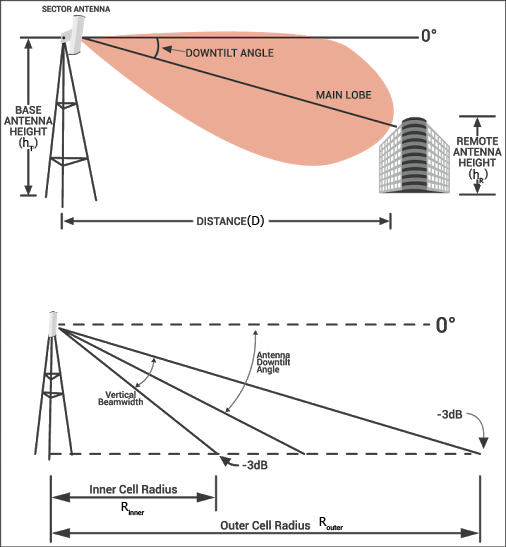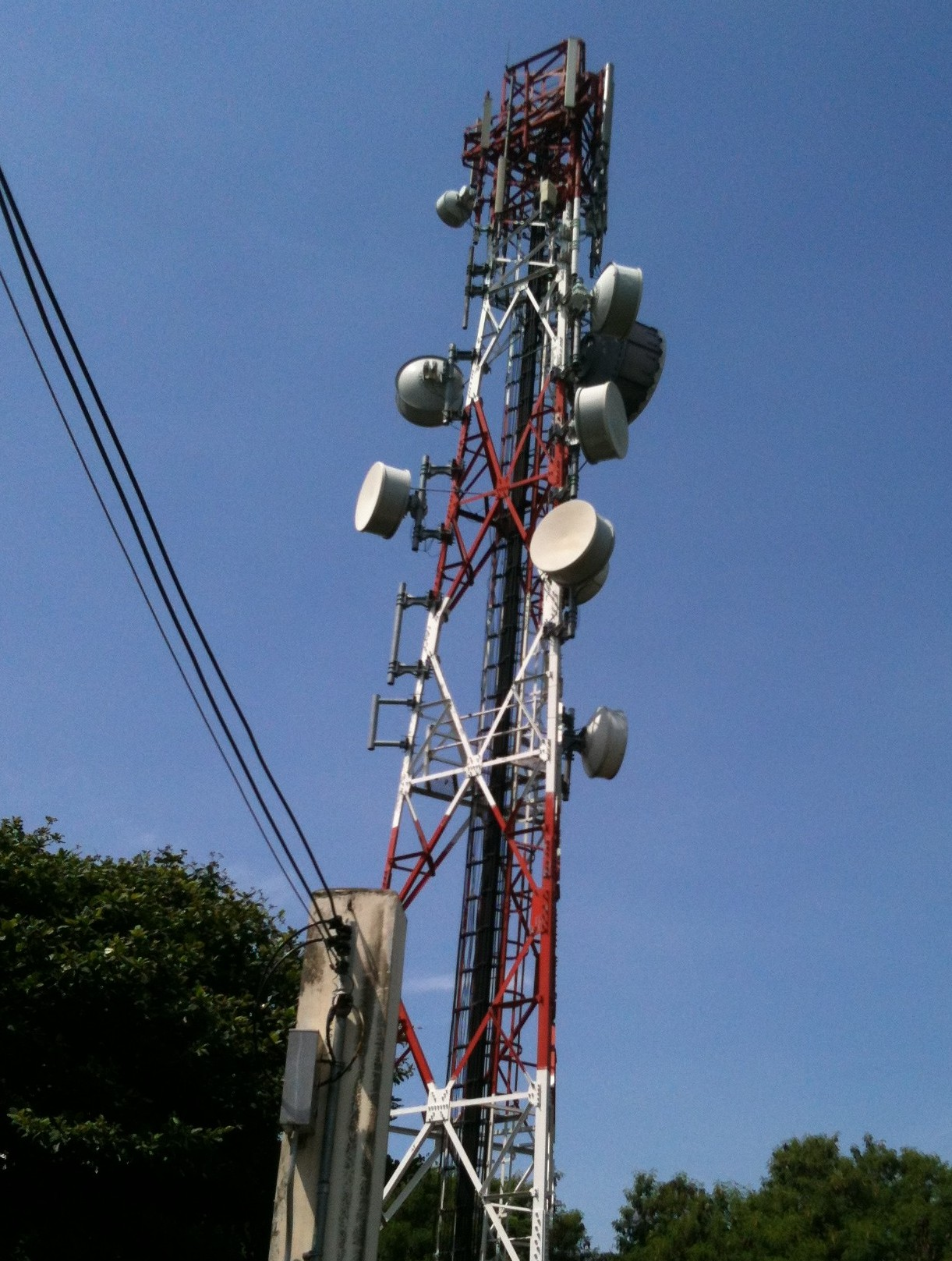# Antenna Downtilt and Coverage Calculator

## This calculator will determine the correct antenna downtilt angle given the heights of the antennas and distance between them.

° (degree)
ft (feet)
ft (feet)
ft (feet)

### Overview

This calculator will help you determine the approximate downward angle (degrees) for a transmitting antenna in order to propagate signals with optimal strength and coverage. Besides the downward angle, this tool will also give you the inner and outer radii of coverage given the antenna's beamwidth.### Equation

$$A_{DT} = tan^{-1}(\frac{h_{T}-h_{R}}{D})$$

$$R_{outer} = \frac{h_{T}-h_{R}}{tan(A_{DT}-\frac{\theta_{BW})}{2}}$$

$$R_{inner} = \frac{h_{T}-h_{R}}{tan(A_{DT}+\frac{\theta_{BW})}{2}}$$

Where:

$$A_{DT}$$ = antenna downtilt in degrees

$$h_{T}$$ = height of the transmitting antenna

$$h_{R}$$ = height of the receving antenna

$$D$$ = distance between antennas

$$R_{outer}$$ = outer radius of coverage

$$R_{inner}$$ = inner radius of coverage

$$\theta_{BW}$$ = beamwidth of the antenna in degrees

### Applications

Network engineers often need to determine the correct antenna downtilt for maximum signal strength. In fact, the efficiency of a cellular network relies heavily on the adjustment of the transmit and receive antennas. Adjusting the antenna's tilt is one of the more important system optimization tasks that is carried out by a specialized team. Note that engineers are mostly concerned with "down" tilt and not "up" tilt; this is because antennas are mostly pointing towards the earth (unless they are communicating with a satellite).Besides cellular networks, antenna downtilt calculations are also needed in Land Mobile Radio (LMR), distributed antennas systems (DAS), and broadcast communications.